top of page
Search

# Operators Blocks (Part 1)

Updated: Dec 16, 2021

## What operators blocks are?

These blocks allow to script math equations and string handling. These blocks are applicable for sprites and backdrops. Also, these blocks must be used with other blocks like control blocks.## How to use “() + ()” block?

This block allows to add two numbers. This block must be used with other blocks.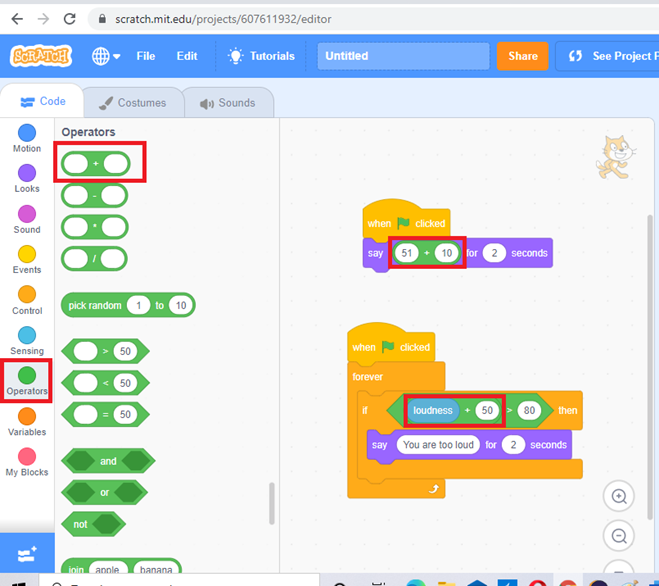## How to use “() – ()” block?

This block allows to takeaway a number from another. This block must be used with other blocks.## How to use “() * ()” block?

This block allows to multiply two numbers. This block must be used with other blocks.## How to use “() / ()” block?

This block allows to divide numbers. This block must be used with other blocks.## How to use “Pick random () to ()” block?

The block returns a random number ranging from the first given number to the second, including both endpoints. You can use positive numbers (example 10 to 30), negative numbers (example -20 to – 40), integers (example 5 to 15) and float (example 0.1 to 0.5)## How to use “() > ()” block?

This block allows to check the first number is greater than the second number.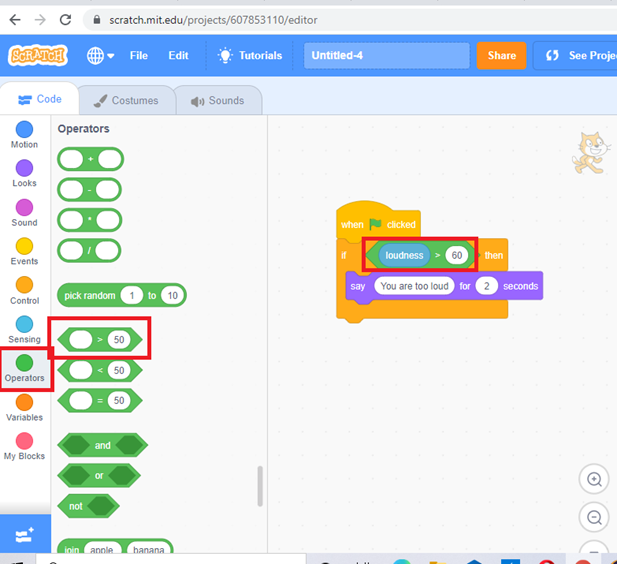## How to use “() < ()” block?

This block allows to check the first number is less than the second number.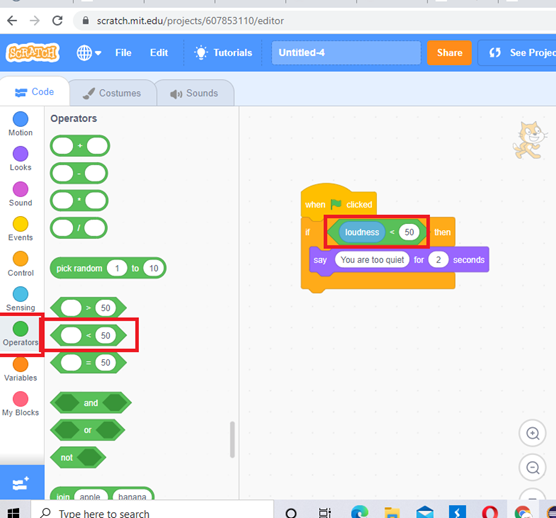## How to use “() = ()” block?

This block allows to check first number or string is equal to second number or string.## How to use “() and ()” block?

When both conditions are true the instructions under the conditions will be executed.## How to use “() or ()” block?

When either condition 1 or condition 2 is true the instructions under the conditions will be executed.## How to use “not ()” block?

This block allows to check the condition is not true. When the condition is not true the instructions under the condition will be executed.## How to use “join () ()” block?

This block allows to join two strings.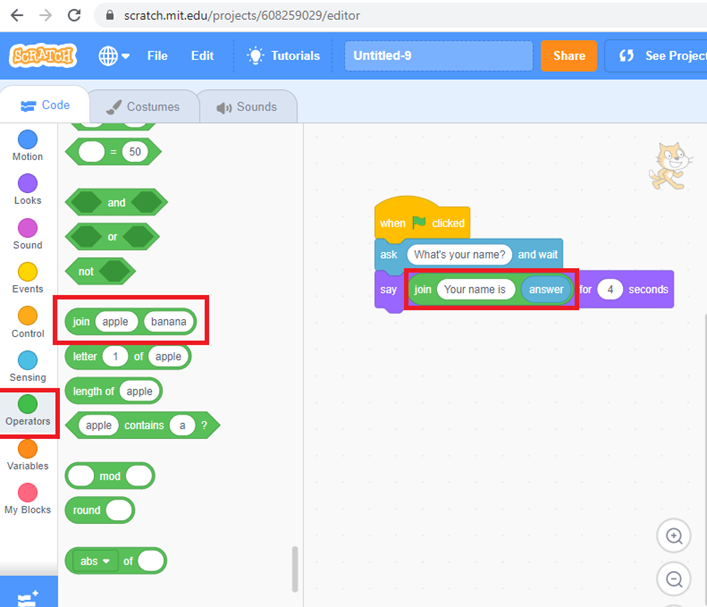## How to use “letter () of ()” block?

This block allows to get the specific letter in a string.## How to use “length of ()” block?

This block allows to find the length of the string.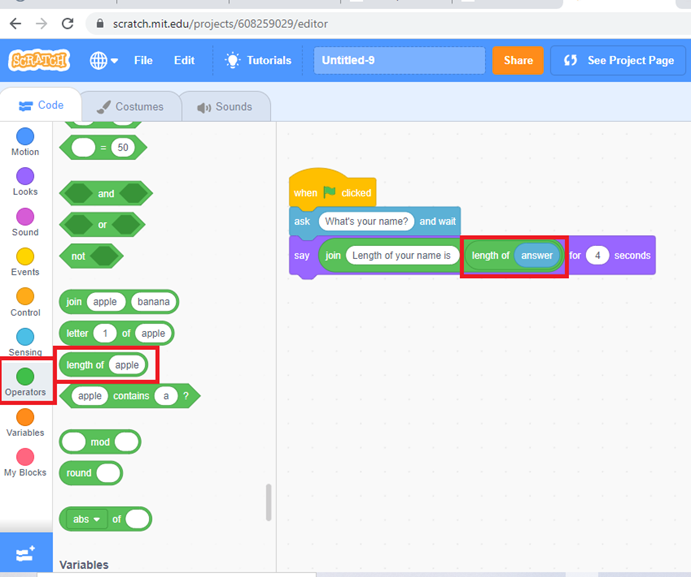## How to use “() contains ()?” block?

This block allows to check a specific letter presents in the given string.## How to use “() mod ()” block?

The mod is the remainder after dividing one number by another. This block allows to find the mod of two numbers.## How to use “round ()” block?

The block rounds the given number to the nearest integer. It follows the standard rules of rounding; decimals that are .5 or higher are rounded up, whereas decimals less than .5 are rounded down.## Exercise 11

1. What are the mathematical operators you learnt so far? Which one you like the most?